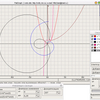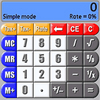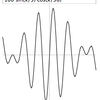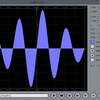DeadLine is a free program useful for solving equations, plotting graphs and obtaining an in-depth analysis of a function. Designed especially for students and engineers, the freeware combines graph plotting with advanced numerical calculus, in a very intuitive approach. Most equations are supported, including algebraic equations, trigonometric equations, exponential equations, parametric equations. DeadLine solves equations graphically and numerically. It displays the graph of the function and a list of the real roots of the equation. You can evaluate the function and the first two derivatives, find extrema of the function and integrate numerically. You can even obtain the first two derivatives. Save the results provided by DeadLine so you can easily integrate in your school projects. While there is no flawless method for solving equations, the program combines the most successful methods in order to deliver you the right answer. No more problems on getting your homework done. Meet th

## Related software (5)## FlatGraph

Plotting functions (usual and parametric) with more possibilities. Differentiation of any order (with simplification). Construction of tangents to the graph. The simple and clear i ...## PalmaryCalc

PalmaryCalc from a simple to a scientific calculator with different input methods, conversion functions, credit, base number conversions, integer math calculations , logical and bi ...## Functions

Visualizes and studies functions of one variable to find roots, extrema, integral, derivatives, graph. Results can be saved or printed. You can copy the graph to the clipboard. You ...## Jep Java

Jep is a Java library for parsing and evaluating mathematical expressions. With this package you can allow your users to enter an arbitrary formula as a string, and instantly evalu ...## WinDraw

This software utility can plot regular or parametric functions, in Cartesian or polar coordinate systems, and is capable to evaluate the roots, minimum and maximum points as well a ...